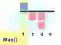# Python max, min Examples

Call the max and min built-in functions to search for the largest and smallest elements in lists.

Max, min. In a list, we do not need to search for the largest or smallest element. Instead we use max and min. The maximum or minimum value is returned.Argument details. With 2 arguments, max returns the larger of the two. And min returns the smaller. We can use max and min to compare two variables.

List example. Max and min act on iterables. A list is a common iterable. We use max and min on a list with positive and negative integer elements.List, strings
Here: Max returns the value 1000, which is larger than all other elements in the list. And min returns negative 100.
Tip: In all uses, max and min return a single value. If all elements are equal, that value is returned.Python program that uses max, min values = [1, -1, -2, 0] # Find the max and min elements. print(max(values)) print(min(values)) Output 1 -2

Strings. Consider the use of max and min on a list of strings. An alphabetic sort is used to test strings. The same comparison is used to sort a list.
So: The string that is sorted first is the min. And the string that comes last in sorting is the max.
Python program that uses max, min on string list values = ["cat", "bird", "apple"] # Use max on the list of strings. result = max(values) print("MAX", values, result) # Use min. result = min(values) print("MIN", values, result) Output MAX ['cat', 'bird', 'apple'] cat MIN ['cat', 'bird', 'apple'] apple

Two arguments. A program has two variables. One has a value larger than the other. We can determine the larger value by passing both variables to max.
Java: This use of max and min is similar to methods like Math.max in Java and Math.Max in C#.
Python program that uses max, min with two arguments value1 = 100 value2 = -5 # Call max with two arguments. # ... The larger argument is returned. maximum = max(value1, value2) print("MAX", maximum) # Call min with two arguments. minimum = min(value1, value2) print("MIN", minimum) # If the arguments are equal, max and min return that value. print("MAX", max(0, 0)) print("MIN", min(0, 0)) Output MAX 100 MIN -5 MAX 0 MIN 0

A summary. With max and min, we search iterables for the largest and smallest elements. We can use these built-in functions to compare 2 arguments and return the larger or smaller one.

Home

© 2007-2020 sam allen. send bug reports to info@dotnetperls.com.